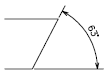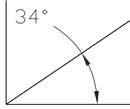# DIMANGULAR (Command)

Creates an angular dimension.

Find

Measures the angle between selected geometric objects or 3 points.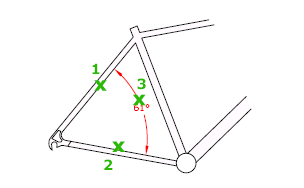The following prompts are displayed.

Arc Selection

Uses points on the selected arc or polyline arc segment as the defining points for a three-point angular dimension. The center of the arc is the angle vertex. The arc endpoints become the origin points of the extension lines.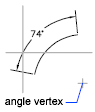The dimension line is drawn as an arc between the extension lines. The extension lines are drawn from the angle endpoints to the intersection of the dimension line.

Circle Selection

Uses the selection point as the origin of the first extension line. The center of the circle is the angle vertex.

The second angle endpoint is the origin of the second extension line and does not have to lie on the circle.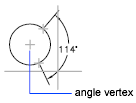Line Selection

Defines the angle using two lines or polyline segments.

The program determines the angle by using each line as a vector for the angle and the intersection of the lines for the angle vertex. The dimension line spans the angle between the two lines. If the dimension line does not intersect the lines being dimensioned, extension lines are added as needed to extend one or both lines. The arc is always less than 180 degrees.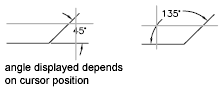Three-Point Specification

Creates a dimension based on three points you specify.

The angle vertex can be the same as one of the angle endpoints. If you need extension lines, the endpoints are used as origin points.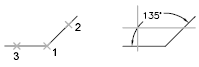The dimension line is drawn as an arc between the extension lines. The extension lines are drawn from the angle endpoints to the intersection of the dimension line.

Dimension Arc Line Location

Specifies the placement of the dimension line and determines the direction to draw the extension lines.

Mtext

Displays the In-Place Text Editor, which you can use to edit the dimension text. To add a prefix or a suffix, enter the prefix or suffix text before or after the generated measurement. Use control codes and Unicode character strings to enter special characters or symbols. See Control Codes and Special Characters.

The current dimension style determines the appearance of the generated measurements.

Text

Customizes the dimension text at the Command prompt. The generated dimension measurement is displayed within angle brackets.

To include the generated measurement, use angle brackets (< >) to represent the generated measurement.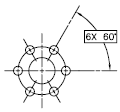Dimension text properties are set on the Text tab of the New, Modify, and Override Dimension Style dialog boxes.

Angle

Changes the angle of the dimension text.